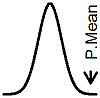P.Mean: When should I use the Fisher's Exact Test and when should I use the Chi-Square Test (created 2012-09-19). News: Sign up for "The Monthly Mean," the newsletter that dares to call itself average, www.pmean.com/news.Dear Professor Mean, I was running crosstabs in SPSS for a two-by-two table and the p-values disagree. The p-value for the Pearson Chi-Square is 0.04 and the p-value for the Fisher's Exact Test (2-sided) is 0.06. Which one should I use?

Well, you're not done yet. The goal of any data analysis is to run at least ten tests and then choose the one with the smallest p-value.

No, no, I'm just kidding.

In a perfect world, you would have specified the test statistic that you would use in the original research protocol. But most protocols fail to specify this detail, so you need to come up with a post hoc justification for your choice. The post hoc justification, of course, should not be based on whether the test is statistically significant or not. It should be based on a criteria that is unrelated to the p-value itself. Thankfully, there is such a criteria, and it is based on the expected cell counts.

Here's a schematic layout of the two by two table with totals added at the marginsThe Chi-Square test is an approximate test and it usually works pretty well. The situations where it is not a good approximation are when the expected cell counts are small. The expected counts relate to the row and column totals. Here's the formula.where the subscripts i and j refer to the particular row and column of the table respectively. R and C refer to the corresponding row and column totals and N is the total sample size.

You don't have to calculate these values to spot a case where the expected counts are small. If one of the row or column totals is very small, then at least one of your expected counts will be very small also, because the expected counts have to produce the same row and column totals as the observed counts do.

Also, most packages will keep you informed about small expected cell counts. Here's an example of a footnote in the SPSS CROSSTABS procedure.The rule of thumb is that the Chi-Square Test is a good approximation whenever all of the expected cell counts are larger than 5.0. Some people have argued that the Chi-Square Test is fine as long as all of the expected cell counts are larger than 1.0. If you are looking at something larger than a two by two table, then you might see a mention that a certain percentage of the expected counts need to be larger than 5.0.

If you look at the formula for the Chi-Square Test, you can get a hint at why small expected counts are a problem.Notice that the expected count appears in the denominator. If the expected count is small, then that term will dominate the calculations. The test statistic will be highly sensitive to small absolute changes in the expected count.

I, myself, am don't look carefully at expected counts. I am pretty conservative on this issue. Anytime that any of the row or column totals is anywhere close to small, I just prefer the Fisher's Exact Test. Why not? It works for expected counts of 0.5. It works for expected counts of 2.5. It works for expected counts of 25. The only thing stopping you is that Fisher's Exact Test takes a lot of time to compute. With today's computers, that's not a serious limitation even when your row and column totals get into the hundreds.

For me, I'll take the Fisher's Exact p-value whenever any of the row or column totals is in the low double digits.This page was written by Steve Simon and is licensed under the Creative Commons Attribution 3.0 United States License. Need more information? I have a page with general help resources. You can also browse for pages similar to this one at Hypothesis Testing.